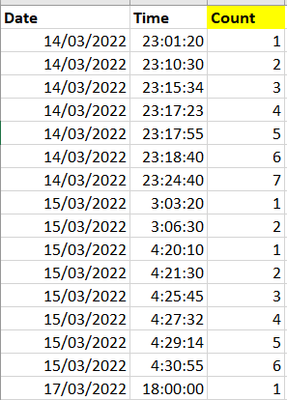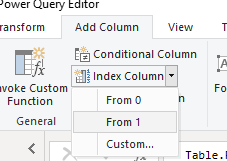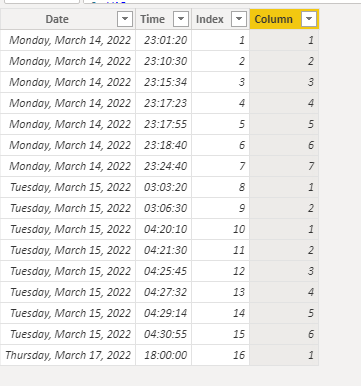cancel
Showing results for
Did you mean:Frequent Visitor

## How to write specific time formula

If the next row is in timespan within 30 minutes of the current row then increment count +1 else reset count.

Below you can see the desired output:1 ACCEPTED SOLUTIONCommunity Support

Hi @Niels_IRD ,Then create a calculated column:

``````Column =
VAR _a =
CALCULATE (
MAX ( 'Table'[Date] ) + MAX ( 'Table'[Time] ),
FILTER ( 'Table', [Index] = EARLIER ( 'Table'[Index] ) - 1 )
)
VAR _b = [Date] + [Time]
VAR _c =
'Table',
"a",
CALCULATE (
MAX ( 'Table'[Date] ) + MAX ( 'Table'[Time] ),
FILTER ( 'Table', [Index] = EARLIER ( 'Table'[Index] ) - 1 )
),
"b", [Date] + [Time]
)
VAR _diff =
ADDCOLUMNS ( _c, "Diff", DATEDIFF ( [a], [b], MINUTE ) )
VAR _maxindex =
MINX (
FILTER ( _diff, [Diff] > 30 && [Index] >= EARLIER ( 'Table'[Index] ) ),
[Index]
)
VAR _minindex =
MAXX (
FILTER ( _diff, [Diff] > 30 && [Index] <= EARLIER ( 'Table'[Index] ) ),
[Index]
) + 0
VAR _filtertable =
FILTER ( 'Table', [Index] >= _minindex && [Index] <= _maxindex )
VAR _count =
COUNTROWS ( FILTER ( _filtertable, [Index] <= EARLIER ( 'Table'[Index] ) ) )
RETURN
_count
``````

Final output:Best Regards,

Jianbo Li

If this post helps, then please consider Accept it as the solution to help the other members find it more quickly.

2 REPLIES 2Community Support

Hi @Niels_IRD ,Then create a calculated column:

``````Column =
VAR _a =
CALCULATE (
MAX ( 'Table'[Date] ) + MAX ( 'Table'[Time] ),
FILTER ( 'Table', [Index] = EARLIER ( 'Table'[Index] ) - 1 )
)
VAR _b = [Date] + [Time]
VAR _c =
'Table',
"a",
CALCULATE (
MAX ( 'Table'[Date] ) + MAX ( 'Table'[Time] ),
FILTER ( 'Table', [Index] = EARLIER ( 'Table'[Index] ) - 1 )
),
"b", [Date] + [Time]
)
VAR _diff =
ADDCOLUMNS ( _c, "Diff", DATEDIFF ( [a], [b], MINUTE ) )
VAR _maxindex =
MINX (
FILTER ( _diff, [Diff] > 30 && [Index] >= EARLIER ( 'Table'[Index] ) ),
[Index]
)
VAR _minindex =
MAXX (
FILTER ( _diff, [Diff] > 30 && [Index] <= EARLIER ( 'Table'[Index] ) ),
[Index]
) + 0
VAR _filtertable =
FILTER ( 'Table', [Index] >= _minindex && [Index] <= _maxindex )
VAR _count =
COUNTROWS ( FILTER ( _filtertable, [Index] <= EARLIER ( 'Table'[Index] ) ) )
RETURN
_count
``````

Final output:Best Regards,

Jianbo Li

If this post helps, then please consider Accept it as the solution to help the other members find it more quickly.Super User

@Niels_IRD ,a new column

countx(filter(Table, [Date] =earlier([Date]) && [Time] <= earlier([Time]) ), [Date])